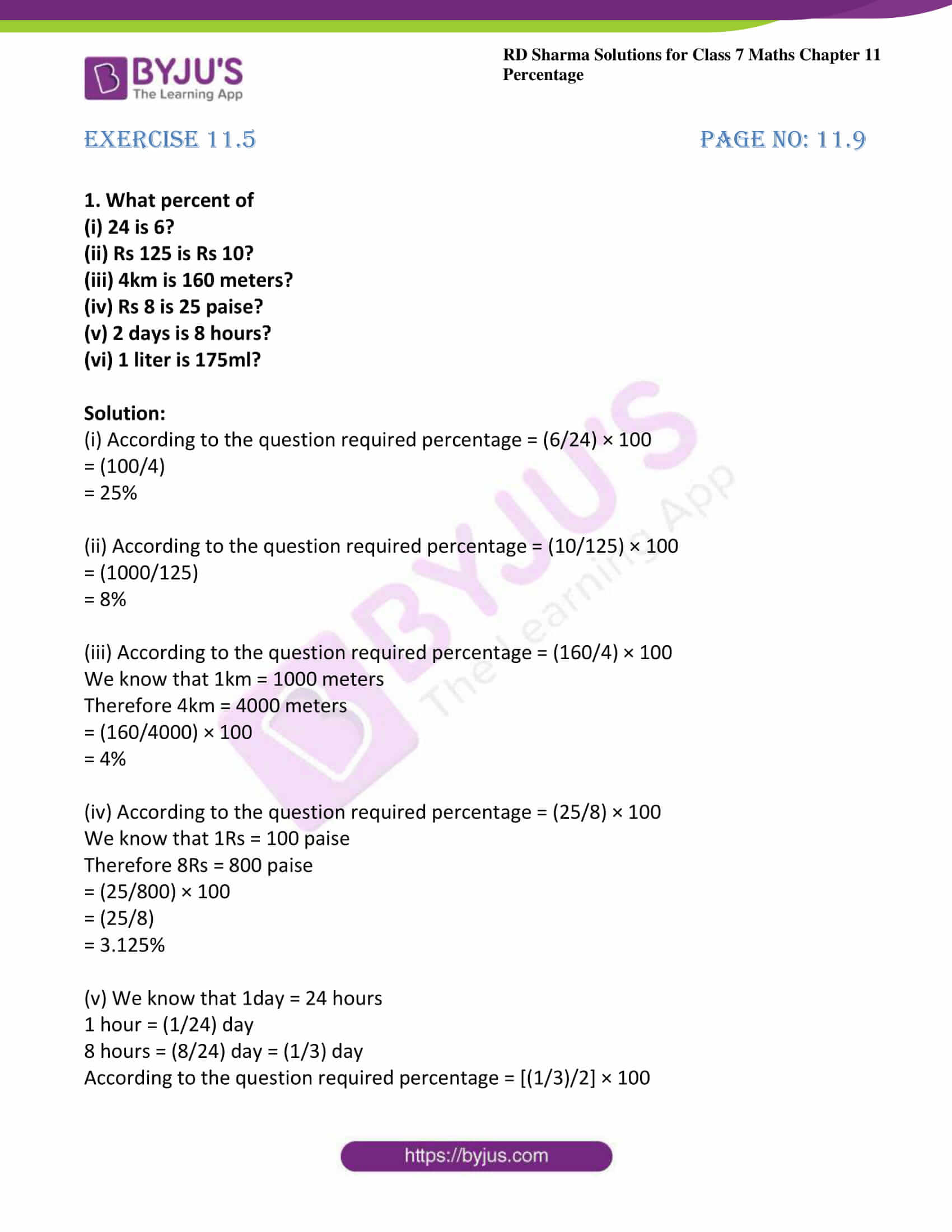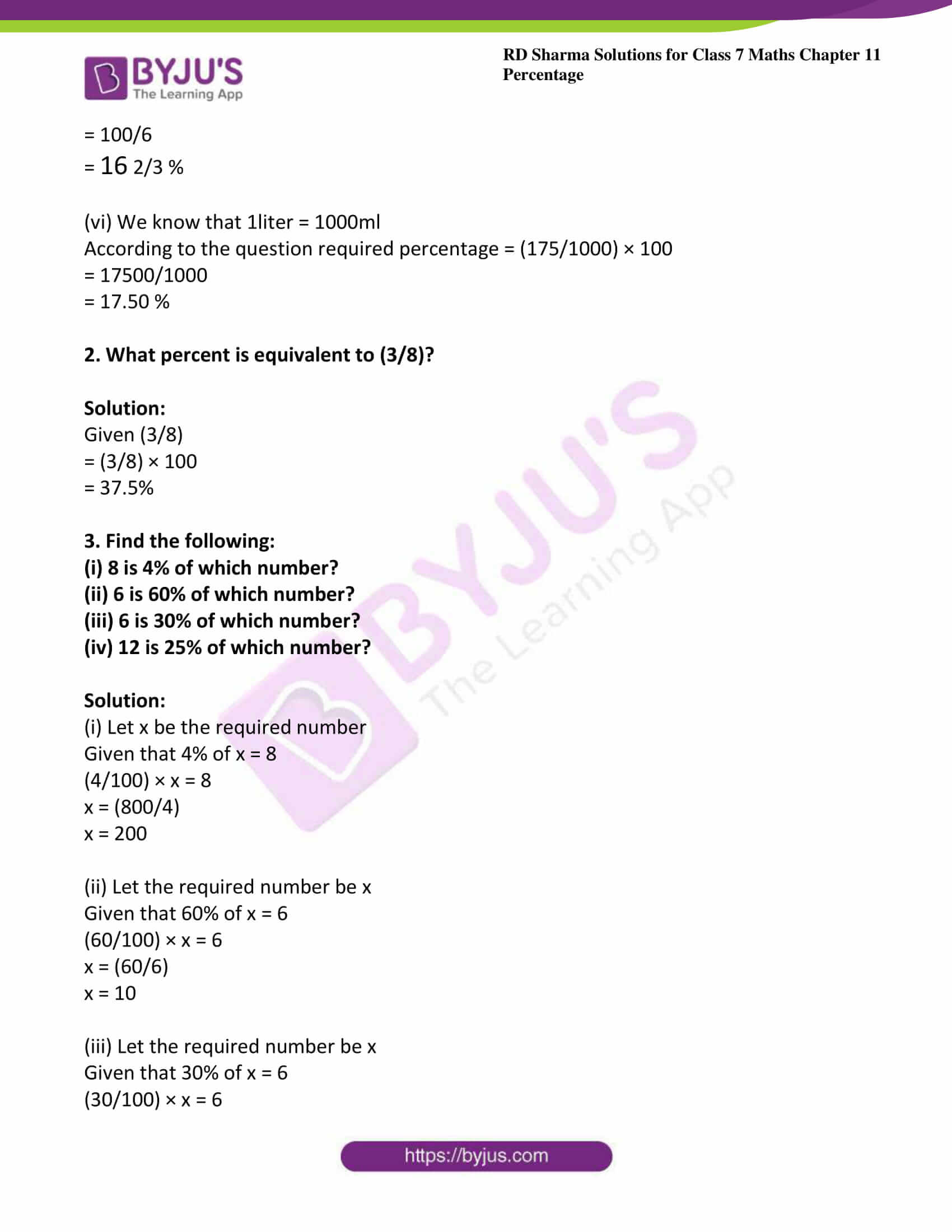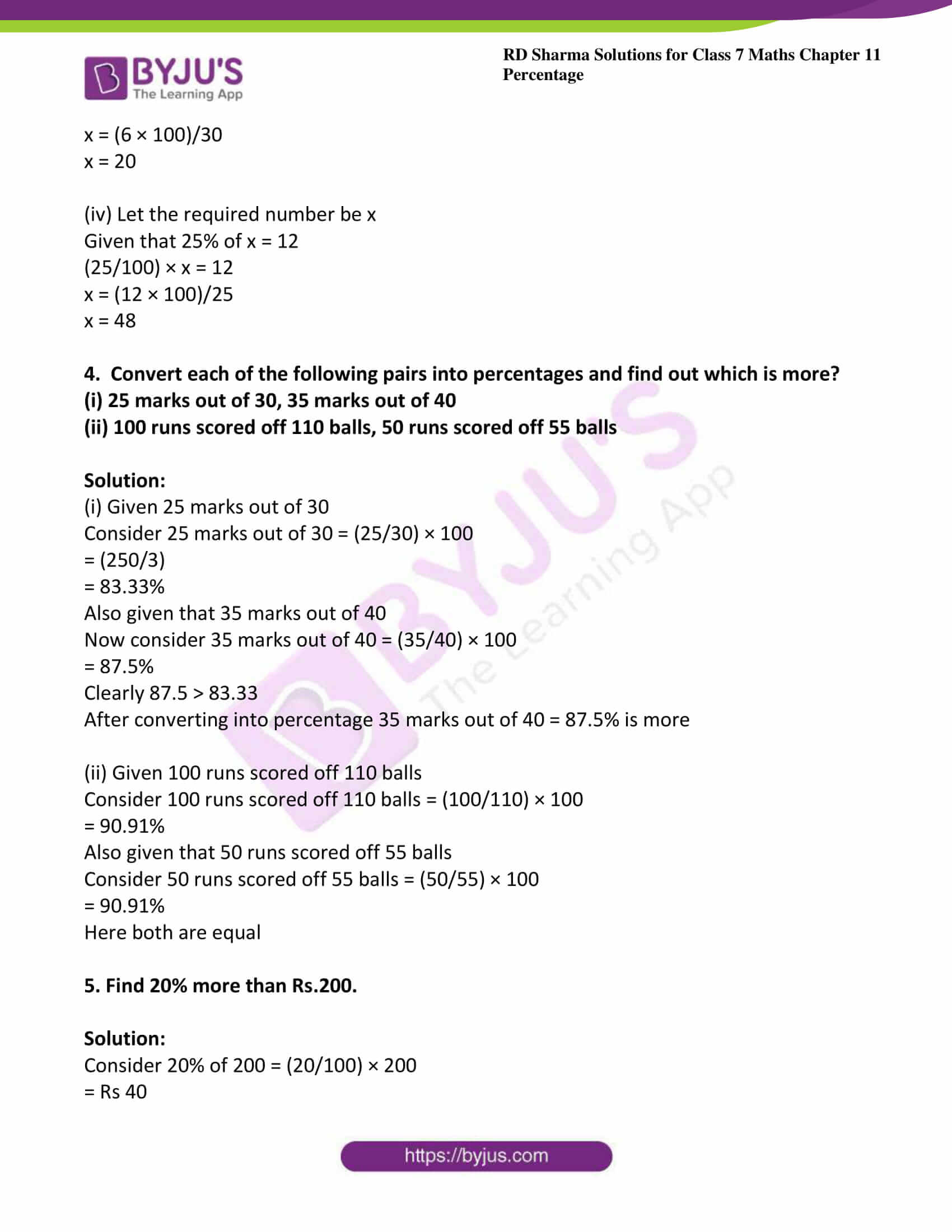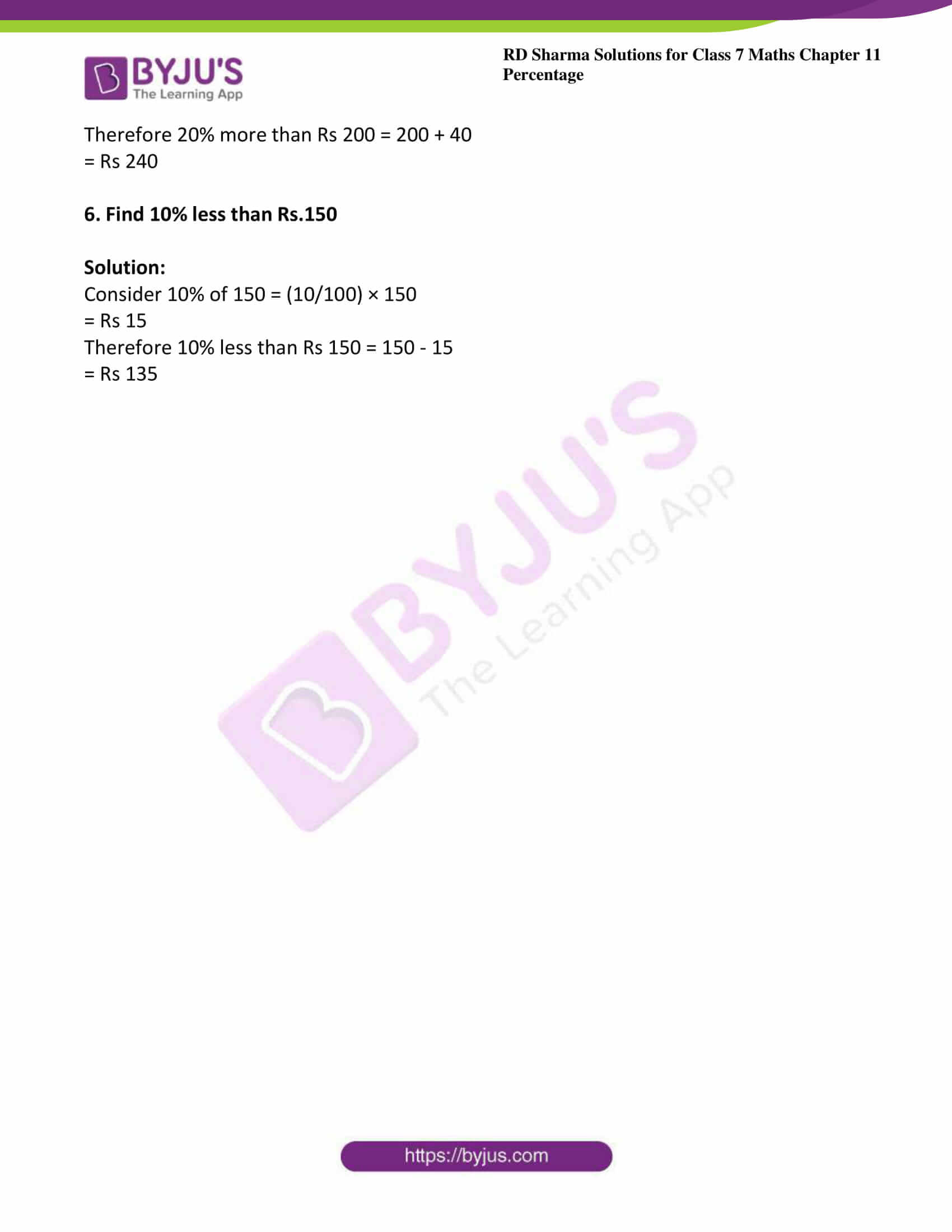# RD Sharma Solutions For Class 7 Maths Chapter 11 Percentage Exercise 11.5

The PDF of RD Sharma Solutions for Exercise 11.5 of Class 7 Maths Chapter 11 Percentage are provided here. The questions present have been solved by BYJU’S experts in Maths, and this will help students solve the problems without any difficulties. This exercise contains six questions with many sub-questions. By practising sincerely, the students can obtain worthy results in exams using the RD Sharma Solutions for Class 7. This exercise deals with comparing two quantities and finding what percent of one quantity is of the other quantity.

## Download the PDF of RD Sharma Solutions For Class 7 Maths Chapter 11 – Percentage Exercise 11.5### Access answers to Maths RD Sharma Solutions For Class 7 Chapter 11 – Percentage Exercise 11.5

Exercise 11.5 Page No: 11.9

1. What percent of

(i) 24 is 6?

(ii) Rs 125 is Rs 10?

(iii) 4km is 160 meters?

(iv) Rs 8 is 25 paise?

(v) 2 days is 8 hours?

(vi) 1 liter is 175ml?

Solution:

(i) According to the question required percentage = (6/24) × 100

= (100/4)

= 25%

(ii) According to the question required percentage = (10/125) × 100

= (1000/125)

= 8%

(iii) According to the question required percentage = (160/4) × 100

We know that 1km = 1000 meters

Therefore 4km = 4000 meters

= (160/4000) × 100

= 4%

(iv) According to the question required percentage = (25/8) × 100

We know that 1Rs = 100 paise

Therefore 8Rs = 800 paise

= (25/800) × 100

= (25/8)

= 3.125%

(v) We know that 1day = 24 hours

1 hour = (1/24) day

8 hours = (8/24) day = (1/3) day

According to the question required percentage = [(1/3)/2] × 100

= 100/6

= 16 2/3 %

(vi) We know that 1liter = 1000ml

According to the question required percentage = (175/1000) × 100

= 17500/1000

= 17.50 %

2. What percent is equivalent to (3/8)?

Solution:

Given (3/8)

= (3/8) × 100

= 37.5%

3. Find the following:

(i) 8 is 4% of which number?

(ii) 6 is 60% of which number?

(iii) 6 is 30% of which number?

(iv) 12 is 25% of which number?

Solution:

(i) Let x be the required number

Given that 4% of x = 8

(4/100) × x = 8

x = (800/4)

x = 200

(ii) Let the required number be x

Given that 60% of x = 6

(60/100) × x = 6

x = (60/6)

x = 10

(iii) Let the required number be x

Given that 30% of x = 6

(30/100) × x = 6

x = (6 × 100)/30

x = 20

(iv) Let the required number be x

Given that 25% of x = 12

(25/100) × x = 12

x = (12 × 100)/25

x = 48

4. Convert each of the following pairs into percentages and find out which is more?

(i) 25 marks out of 30, 35 marks out of 40

(ii) 100 runs scored off 110 balls, 50 runs scored off 55 balls

Solution:

(i) Given 25 marks out of 30

Consider 25 marks out of 30 = (25/30) × 100

= (250/3)

= 83.33%

Also given that 35 marks out of 40

Now consider 35 marks out of 40 = (35/40) × 100

= 87.5%

Clearly 87.5 > 83.33

After converting into percentage 35 marks out of 40 = 87.5% is more

(ii) Given 100 runs scored off 110 balls

Consider 100 runs scored off 110 balls = (100/110) × 100

= 90.91%

Also given that 50 runs scored off 55 balls

Consider 50 runs scored off 55 balls = (50/55) × 100

= 90.91%

Here both are equal

5. Find 20% more than Rs.200.

Solution:

Consider 20% of 200 = (20/100) × 200

= Rs 40

Therefore 20% more than Rs 200 = 200 + 40

= Rs 240

6. Find 10% less than Rs.150

Solution:

Consider 10% of 150 = (10/100) × 150

= Rs 15

Therefore 10% less than Rs 150 = 150 – 15

= Rs 135